# Class 12 Maths NCERT Solutions for Chapter 6 Application of Derivatives Exercise 6.5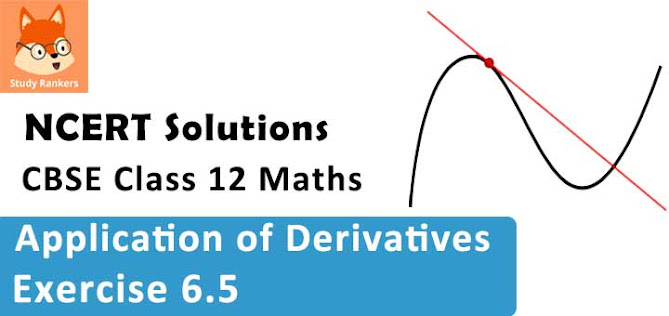### Application of Derivatives Exercise 6.5 Solutions

1. Find the maximum and minimum values, if any, of the following functions given by
(i) f(x) = (2x − 1)2 + 3
(ii) f(x) = 9x2 + 12x + 2
(iii) f(x) = -(x -1)2 + 10
(iv) g(x) = x3 + 1

Solution

(i) The given function is f(x) = (2x - 1)2 + 3.
It can be observed that (2x - 1)2 ≥ 0 for every x ∊ R.
Therefore, f(x) = (2x - 1)2 + 3 ≥ 3 for every x ∊ R.
The minimum value of f is attained when 2x - 1 = 0
2x - 1 = 0
⇒ x = 1/2
∴ Minimum value of f = f(1/2) = [2. (1/2) - 1]2 + 3 = 3
Hence, function f does not have a maximum value.

(ii) The given function is f(x) = 9x2 + 12x + 2 = (3x + 2)2 - 2.
It can be observed that (3x + 2)2 ≥ 0 for every x ∈ R.
Therefore, f(x)  = (3x +2)2 - 2 ≥ -2 for every x ∈ R.
The minimum value of f is attained when 3x + 2 = 0
3x + 2 = 0
⇒ x = -2/3
∴ Minimum value of f =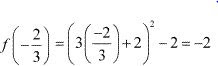Hence, function f does not have a maximum value.

(iii) The given function is f(x) = -(x - 1)2 + 10.
It can be observed that (x - 1)2 ≥ 0 for every x ∈ R.
Therefore, f(x) = -(x - 1)2 + 10 ≤ 10 for every x ∈ R.
The maximum value of f is attained when (x - 1) = 0.
(x - 1) = 0
⇒ x = 1
∴ Maximum value of f = f(1) = -(1 - 1)2 + 10 = 10
Hence, function f does not have a minimum value.

(iv) The given function is g(x) x3 + 1.
Hence, function g neither has a maximum value nor a minimum value.

2. Find the maximum and minimum values, if any, of the functions given by
(i) f(x) = |x + 2| − 1
(ii) g(x) = -|x + 1| + 3
(iii) h(x) = sin(2x) + 5
(iv) f(x)  = |sin 4x + 3|
(v) h(x) = x+  4, x ∊ (-1, 1)

Solution

(i)  f(x) = |x + 2| − 1
We know that |x + 2| ≥ 0 for every x ∊ R.
Therefore, f(x) = |x + 2| - 1 ≥ - 1 for every x ∊ R.
The minimum value of f is attained when |x + 2| = 0 .
|x + 2| = 0
⇒ x = -2
∴ Minimum value of f = f(-2) = |-2 + 2| - 1 = -1
Hence, function f does not have a maximum value.

(ii) g(x) = -|x + 1| + 3
We know that -|x + 1|≤ 0 for every x ∊  R
Therefore, g(x) = -|x + 1| + 3≤ 3 for every x ∊ R.
The maximum value of g is attained when |x + 1| = 0.
|x + 1| = 0
⇒ x = -1
∴ Maximum value of g = g(-1) = -|-1 + 1| + 3 = 3
Hence, function g does not have a minimum value.

(iii) h(x) = sin 2x + 5
We know that - 1 ≤ sin 2x ≤ 1.
⇒ -1 + 5 ≤ sin 2x + 5 ≤ 1 + 5
⇒ 4 ≤ sin 2x + 5 ≤ 6
Hence, the maximum and minimum values of h are 6 and 4 respectively.

(iv) f(x) = |sin 4x + 3|
We know that -1 ≤ sin 4x ≤ 1.
⇒ 2 ≤sin 4x + 3 ≤4
⇒ 2 ≤ |sin 4x + 3| ≤ 4
Hence, the maximum and minimum values of f are 4 and  2 respectively.

(v) h(x) = x + 1, x ∊ (-1, 1)
Here, if a point x0 is closest to -1, then we find x0/2 + 1 < x0 + 1 for all x0 ∈ (-1, 1).
Also, if x1 is closest to 1, then x1 + 1 < (x1 + 1)/2 + 1 for all x1 ∊ (-1, 1).
Hence, function h(x) has neither maximum nor minimum value in (-1, 1).

3. Find the local maxima and local minima, if any, of the following functions. Find also the local maximum and the local minimum values, as the case may be
(i) f(x) = x2
(ii) g(x) = x3 - 3x
(iii) h(x) = sin x + cos x, 0 < x < π/2
(iv) f(x) = sin x - cos x, 0 < x < 2π
(v) f(x) = x3 - 6x2  + 9x + 15
(vi) g(x) = x/2 + 2/x , x > 0
(vii) g(x) = 1/(x2 + 2)
(viii) f(x) = x√(1- x) , 0 < x < 1

Solution

(i) f(x) = x2
∴ f '(x) = 2x
Now,
f '(x) = 0
⇒ x = 0
Thus, x = 0 is the only critical point which could possible be the point of local maxima or local minima of f.
We have f''(0) = 2, which is positive.
Therefore, by second derivative test, x = 0 is a point of local minima and local minimum value of f at x = 0 is f(0) = 0.

(ii) g(x) = x3 - 3x
∴ g '(x) = 3x2 - 3
Now,
g''(x) = 0
⇒ 3x2 = 3
⇒ x = ± 1
g''(x) = 6x
g''(1) = 6 > 0
g''(-1) = -6 < 0
By second derivative test, x = 1 is a point of local minima and local minimum value of g at x = 1 is g(1) = 13 - 3 = 1 - 3= -2. However,
x = -1 is a point of local maxima and local maximum value of g at
x = -1 is g(-1) = (-1)3 - 3(-1) = -1 + 3 = 2.

(iii) h(x) = sin x + cos x, 0 < x < π/2Therefore, by second derivative test, x = π/4 is a point of local maxima and the local maximum value of h at x = π/4 is h(π/4) = sin . (π/4) + cos.(π/4) = 1/√2 + 1/√2 = √2.

(iv) f(x) = sin x - cos x, 0 < x < 2π
∴ f '(x) = cos x + sin x
f '(x) = 0
⇒ cos x = -sin x
⇒ tan x = -1
⇒ x = 3π/4, 7π/4 ∊ (0, 2π)
f ''(x) = - sin x + cos x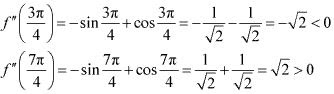Therefore, by second derivative test, x = 3π/4 is a point of local maxima and the local maximum value of f at x = 3π/4 is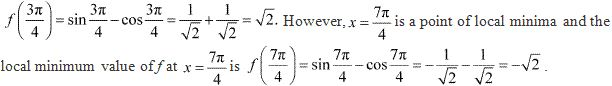(v) f(x) = x3 - 6x2 + 9x + 15
∴ f '(x) = 3x2 - 12x + 9
f 'x = 0 ⇒ 3(x2 - 4x + 3) = 0
⇒ 3(x - 1)(x - 3) = 0
⇒ x = 1, 3
Now, f ''(x) = 6x - 12 = 6(x - 2)
f ''(1) = 6(1 - 2) = -6 < 0
f ''(3) = 6(3 - 2) = 6 > 0
Therefore, by second derivative test, x = 1 is a point of local maxima and the local maximum value of f at x = 1 is f(1) = 1 - 6 + 9 + 15 = 19. However, x = 3 is a point of local minima and the local minimum value of f at x = 3 is f(3) = 27 - 54 + 27 + 15 = 15.

(vi) g(x)  = x/2 + 2/x, x > 0
∴ g'(x) = 1/2 - 2/x2
Now,
g'(x) = 0 gives 2/x2 = 1/2
⇒ x2 = 4
⇒ x = ± 2
Since x > 0, we take x = 2.
Now, g''(x) = 4/x3
g''(2) = 4/23 = 1/2 > 0
Therefore, by second derivative test, x = 2 is a point of local minima and the local minimum value of g at x = 2 is g(2) = 2/2 + 2/2 = 1 + 1 = 2.

(vii) g(x) = 1/(x2 + 2)Now, for values close to x = 0 and to the left of 0, g'(x) > 0. Also, for values close to x = 0 and to the right of 0, g'(x) < 0.
Therefore, by first derivative test, x = 0 is a point of local maxima and the local maximum value of g(0) is 1/(0+2) = 1/2.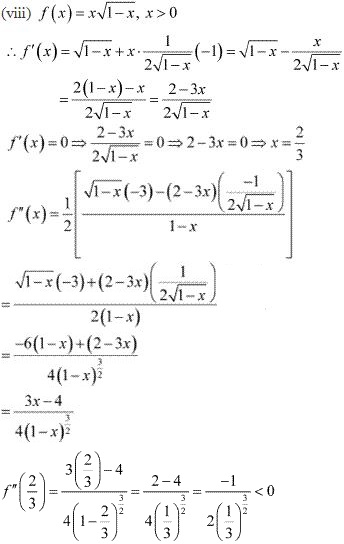Therefore, by second derivative test, x = 2/3 is a point of local maxima and the local maximum value of f at x = 2/3 is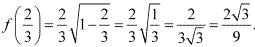4. Prove that the following functions do not have maxima or minima :
(i) f(x) = ex
(ii) g(x) = log x
(iii) h(x) = x3 + x2 + x + 1
Solution
(i)  We have,
f(x) = ex
∴ f '(x) = ex
Now, if f '(x) = 0, then ex = 0 . But, the exponential function can never assume 0 for any value of x.
Therefore, there does not exist c ∊ R  such that f '(c) = 0 .
Hence, function f does not have maxima or minima.

(ii) We have,
g(x) = log x
∴ g'(x) = 1/x
Since log x is defined for a positive number x, g'(x)  > 0 for any x.
Therefore, there does not exist c ∊ R such that g'(c) = 0
Hence, function g does not have maxima or minima.

(iii) We have,
h(x) = x3 + x2 + x+  1
∴ h'(x) = 3x2 + 2x + 1
Now,
h(x) = 0 ⇒ 3x2 + 2x + 1 = 0
⇒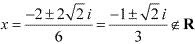Therefore, there does not exist c ∈ R such that h'(c) = 0 .
Hence, function h does not have maxima or minima.

5. Find the absolute maximum value and the absolute minimum value of the following functions in the given intervals :
(i) f(x) = x3 , x ∊ [-2, 2]
(ii) f(x) = sin x + cos x , x ∊ [0, π]
(iii) f(x) = 4x - (1/2)x2 , x ∊ [-2, 9/2]
(iv) f(x) = (x - 1)2 + 3, x ∊ [-3, 1]
Solution
(i) The given function is f(x) = x3 .
∴ f '(x) = 3x2
Now,
f '(x) = 0 ⇒ x = 0
Then, we evaluate the value of f at critical point x = 0 and at end points of the interval [-2, 2].
f(0) = 0
f(-2) = (-2)3 = -8
f(2) = (2)3 = 8
Hence, we can conclude that the absolute maximum value of f on [-2, 2] is 8 occurring at x = 2 .
Also, the absolute minimum value of f on [-2, 2] is -8 occurring at x = -2.

(ii) The given function is f(x) = sin x + cos x
∴ f '(x) = cos x - sin x
Now,
f '(x) = 0 ⇒ sin x = cos x ⇒ tan x = 1 ⇒ x = π/4
Then, we evaluate the value of f at critical point x = π/4 and at the end points of the interval (0, π).
f(π/4) = sin(π/4)+ cos(π/4) = 1/√2 + 1/√2 = 2/√2 = √2
f(0) = sin 0 + cos 0 = 0 + 1 = 1
f(π) = sin π+ cosπ = 0 - 1 = - 1
Hence, we can conclude that the absolute maximum value of f on (0, π) is √2 occurring at x = π/4 and the absolute minimum value of f on (0, π) is -1 occurring at x = π.

(iii) The given function if f(x) = 4x - (1/2)x2 .
∴ f '(x) = 4 - (1/2) (2x) = 4 - x
Now,
f '(x) = 0 ⇒ x = 4
Then; we evaluate the value of f at critical point x = 4 and at the end points of the interval [-2, 9/2 ].
f(4) = 16 - (1/2)(16) = 16 - 8 = 8
f(-2) = -8 - (1/2) (4) = -8-2= -10
f(9/2) = 4(9/2) - (1/2)(9/2)2 = 18 - 81/8 = 18 - 10.125 = 7.875
Hence, we can conclude that the absolute maximum value of f on [-2, 9/2] is  8 occurring at x = 4 and the absolute minimum value of f on [-2, 9/2] is -10 occurring at x = -2.

(iv) The given function is f(x) = (x-1)2 + 3.
∴ f '(x)  = 2(x - 1)
Now,
f '(x) = 0 ⇒ 2(x - 1) = 0 ⇒ x = 1
Then, we evaluate the value of f at critical point x = 1 and at the end points of the interval (-3, 1).
f(1)  = (1 - 1)2 + 3 = 0 + 3 = 3
f(-3) = (-3 - 1)2 + 3 = 16 + 3 = 19
Hence, we can conclude that the absolute maximum value of f on (-3, 1) is 19 occurring at x = -3 and the minimum value of f on (-3, 1) is 3 occurring at x = 1 .

6. Find the maximum profit that a company can make, if the profit function is given by p(x) = 41 − 72x− 18x2
Solution
The profit function is given as p(x) = 41 - 72x - 182 .
∴ p'(x) = -72 - 36x
p''(x) = -36
Now,
p'(x) = 0 ⇒ x = -72/36 = -2
Also,
p"(-2) = -36 < 0
By second derivative test, x = -2 is the point of local maxima of p.
∴ Maximum profit = p(-2)
= 41 - 72(-2) - 18(-2)2
= 41 + 144 - 72 = 113
Hence, the maximum profit that the company can make is 113 units.

7. Find both the maximum value and the minimum value of 3x4 − 8x3 + 12x2 − 48x + 25 on the interval [0, 3].
Solution
Let f(x) = 3x4 − 8x3 + 12x2 − 48x + 25
∴ f '(x) = 12x3 - 24x2 + 24x - 48
= 12(x3 - 2x2 + 2x - 4)
= 12[x2 (x - 2) + 2(x - 2)]
= 12(x -2)(x2 + 2)
Now, f '(x) = 0 gives x = 2 or x2 + 2 = 0 for which there are no real roots.
Therefore, we consider only x = 2 ∊[0, 3].
Now, we evaluate the value of f at critical point x = 2 and at the end points of the interval [0, 3].
f(2) = 3(16) - 8(8) + 12(4) - 48(2) + 25
= 48 - 64 + 48 - 96 + 25
= - 39
f(0) = 3(0) - 8(0) + 12(0) - 48(0) + 25
= 25
f(3) = 3(81) - 8(27) + 12(9) - 48(3) + 25
=243 - 216 + 108 - 144 + 25 = 16
Hence, we can conclude that the absolute maximum value of f on (0, 3) is 25 occurring at x = 0 and the absolute minimum value of f at (0, 3) is  -39 occurring at x = 2.

8. At what points in the interval [0, 2π], does the function sin 2x attain its maximum value?
Solution
Let f(x) = sin 2x .
∴ f'(x) = 2 cos 2x
Now,
f '(x) = 0 ⇒ cos 2x = 0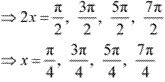Then, we evaluate the values of f at critical points x = π/4, 3π/4, 5π/4, 7π/4 and at the end points of the interval (0, 2π).Hence, we can conclude that the absolute maximum value of f on [0, 2π) is occurring at x = π/4 and x = 5π/4 .

9. What is the maximum value of the function sin x + cos x?
Solution
Let f(x) = sin x + cos x.
∴ f '(x) = cos x - sin x
f '(x) = 0 ⇒ sin x = cos x ⇒ tan x = 1 ⇒ x = π/4, 5π/4 .....,
f "(x) = - sin x - cos x = -(sin x + cos x)
Now, f "(x) will be negative when (sin x + cos x) is positive i.e., when sin x and cos x are both positive. Also, we know that sin x and cos x both are positive in the first quadrant.
Then, f ''(x) will be negative when x ∊ (0, π/2) .
Thus, we consider x = π/4 .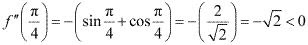∴ By second derivative test, f will be the maximum at x = π/4 and the maximum value of f is (π/4) = sin(π/4) + cos(π/4)  = 1/√2 × 1/√2 = 2/√2 = √2

10. Find the maximum value of 2x3 − 24x + 107 in the interval [1, 3]. Find the maximum value of the same function in [−3, −1].
Solution
Let f(x) = 2x3 − 24x + 107
∴ f '(x) = 6x2 - 24 = 6(x2 - 4)
Now,
f '(x)  = 0 ⇒ 6(x2 - 4) = 0 ⇒ x2 = 4 ⇒ x = ± 2
We first consider the interval (1, 3).
Then, we evaluate the value of f at the critical point x = 2 ∊ (1, 3) and at the end points of the interval (1, 3).
f(2) = 2(8) - 24(2) + 107 = 16 - 48 + 107 = 75
f(1) = 2(1) - 24(1) + 107 = 2 - 24 + 107 = 85
f(3) = 2(27) - 24(3) + 107 = 54 - 72 + 107 = 89
Hence, the absolute maximum value of f(x) in the interval (1, 3) is 89 occurring at x = 3.
Next we consider the interval (-3, -1).
Evaluate the value of f at the critical point x = -2∊ (-3, -1) and at the end points of the interval (1, 3).
f(-3) = 2(-27) - 24(-3) + 107 = -54 + 72 + 107 = 125
f(-1) = 2(-1) - 24(-1) + 107 = -2 + 24 + 107 = 129
f(-2) = 2(-8) - 24(-2) +107 = -16 + 48 + 107 = 139
Hence, the absolute maximum value of f(x) in the interval (-3, -1) is 139 occurring at x = -2

11. It is given that at x = 1, the function x4− 62x2 + ax + 9 attains its maximum value, on the interval [0, 2]. Find the value of a.
Solution
Let f(x) = x4 - 62x2 + ax + 9
∴ f '(x) = 4x3 - 124 x + a
It is given that function f attains its maximum value on the interval (0, 2) at x = 1.
∴ f '(1) = 0
⇒ 4 - 124 + a = 0
⇒ a = 120
Hence, the value of a is 120.

12. Find the maximum and minimum values of x + sin 2x on [0, 2π].
Solution
Let f(x) = x + sin 2x.
∴ f '(x) = 1 + 2 cos 2x
Now, f '(x) = 0 ⇒ cos 2x = -1/2 = - cos (π/3) = cos (π - π/3) = cos (2π/3)
2x = 2nπ ± 2π/3 , n ∊ z
⇒ x = nπ ± π/3, n ∊ z
⇒ x = π/3, 2π/3, 4π/3, 5π/3 ∈ (0, 2π)
Then, we evaluate the value of f at critical points x = π/3, 2π/3, 4π/3, 5π/3 and at the end points of the interval (0, 2π).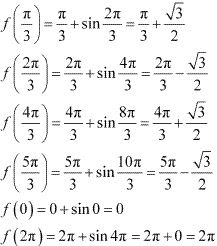Hence, we can conclude that the absolute maximum value of f(x) in the interval (0, 2π) is 2π occurring at x = 2π and the absolute minimum value of f(x) in the interval (0, 2π) is 0 occurring at x = 0.

13. Find two numbers whose sum is 24 and whose product is as large as possible.
Solution
Let one number be x. Then, the other number is (24 - x).
Let p(x) denote the product of the two numbers. Thus, we have :
p(x) = x(24 - x) = 24x - x2
∴ p'(x) = 24 - 2x
p"(x) = -2
Now,
p'(x) = 0 ⇒ x = 12
Also, p"(12) = -2 < 0
∴ By second derivative test, x = 12 is the point of local maxima of P. Hence, the product of the numbers is the maximum when the numbers are  12 and 24 - 12 = 12.

14. Find two positive numbers x and y such that x + y = 60 and xy3 is maximum.
Solution
The two numbers are x and y such that x + y = 60 .
⇒ y = 60 - x
Let f(x) = xy3 .
⇒ f(x) = x (60 - x)3
∴ f '(x) = (60 - x)3 - 3x(60 - x)2
= (60 - x)2 [60 - x -3x]
=(60 - x)2 (60 - 4x)
And, f "(x) = -2(60 - x)(60 - 4x) - 4(60 - x)2
= -2(60 - x)[60 - 4x + 2(60 - x)]
= -2(60 - x)(180 - 6x)
= - 12(60 - x)(30 - x)
Now, f '(x) = 0 ⇒ x = 60 or x  = 15
When x = 60, f "(x) = 0.
When x = 15, f "(x) = -12(60 - 15)(30 - 15) = -12 × 45 × 15 < 0.
∴ By second derivative test, x = 15 is a point of local maxima of f. Thus, function xy3 is maximum when x = 15 and y = 60 - 15 = 45.
Hence, the required numbers are  15 and 45.

15. Find two positive numbers and such that their sum is 35 and the product x2y5 is a maximum
Solution
Let one number be x. Then, the other number is y = (35 - x).
Let p(x) =  x2y5 . Then, we have :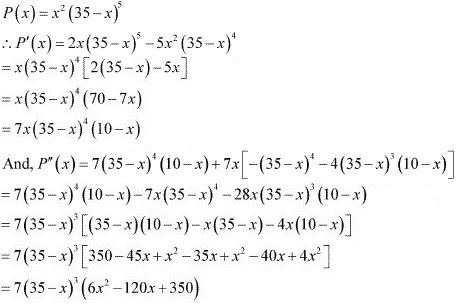Now, P '(x) = 0 ⇒ x = 0, x = 35 , x = 10
When x = 35, P '(x) = p(x) = 0 and y = 35 - 35 = 0. This will make the product x2y5 equal to 0.
When x = 0, y = 35 - 0 = 35 and the product x2y2 will be 0.
∴ x = 0 and x = 35 cannot be the possible values of x.
When x = 10, we have:
P"(x) = 7(35 - 10)3 (6 ×100 - 120 × 10 + 350)
= 7(25)3 (-250) < 0
∴ By second derivative test, P(x) will be the maximum when x = 10 and y =35 - 10 = 25.
Hence, the required numbers are 10 and  25.

16. Find two positive numbers whose sum is 16 and the sum of whose cubes is minimum.
Solution
Let one number be x. Then, the other number is (16 - x).
Let the sum of the cubes of these numbers be denoted by S(x) . Then,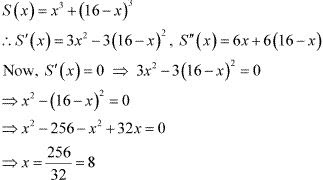Now, S"(8) = 6(8) + 6(16 - 8) = 48 + 48 = 96 > 0
∴ By second derivative test, x = 8 is the point of local minima of S.
Hence, the sum of the cubes of the numbers is the minimum when the numbers are 8 and  16 - 8 = 8.

17. A square piece of tin of side 18 cm is to made into a box without top, by cutting a square from each corner and folding up the flaps to form the box. What should be the side of the square to be cut off so that the volume of the box is the maximum possible?
Solution
Let the side of the square to be cut off be x cm. The, the length and the breadth of the box will be (18 -2x) cm each and the height of the box is x cm.
Therefore, the volume V(x)  of the box is given by,
V(x) = x(18 - 2x)2
∴ V'(x) = (18 - 2x)2 - 4x(18 - 2x)
= (18 - 2x)[18 - 2x - 4x]
= (18 - 2x)(18 - 6x)
= 6 × 2(9 - x)(3 -x)
= 12(9 - x)(3 - x)
And, V"(x) = 12[-(9 - x) - (3 - x)]
= -12(9 - x + 3 - x)
= -12(12 - 2x)
= -24(6x - x)
Now, V'(x) = 0 ⇒ x = 9 or x = 3
If x = 9, then the length and the breadth will become 0.
∴ x ≠ 9.
⇒ x = 3 .
Now, V"(3) = -24(6 - 3) = -72 < 0
∴ By second derivative test, x = 3 is the point of maxima of V.
Hence, if we remove a square of side 3 cm from each corner of the square tin and make a box from the remaining sheet, then the volume of the box obtained is the largest possible.

18. A rectangular sheet of tin 45 cm by 24 cm is to be made into a box without top, by cutting off square from each corner and folding up the flaps. What should be the side of the square to be cut off so that the volume of the box is the maximum possible?
Solution
Let the side of the square to be cut off be x cm. Then, the height of the box is x, the length is 45 - 2x, and the breadth is 24 - 2x.
Therefore, the volume V(x) of the box is given by,
V(x) = x(45 - 2x)(24 - 2x)
= x(1080 - 90x  48x + 4x2 )
= 4x3 - 138x2 + 1080x
∴ V'(x) = 12x2 - 276x + 1080
= 12(x2 - 23x + 90)
= 12(x - 18)(x - 5)
V"(x) = 24x - 276 = 12(2x - 23)
Now, V'(x) = 0 ⇒ x = 18 and x = 5
It is not possible to cut off a square of side 18 cm from each corner of the rectangular sheet.
Thus, x cannot be equal to 18.
∴ x = 5
Now, V"(5) = 12(10 - 23) = 12(-13) = -156 < 0
∴ By second derivative test, x = 5 is the point of maxima.
Hence, the side of the square to be cut off to make the volume of the box maximum possible is 5 cm.

19. Show that of all the rectangles inscribed in a given fixed circle, the square has the maximum area.
Solution
Let a rectangle of length l and breadth b be inscribed in the given circle of radius a.
Then, the diagonal passes through the centre and is of length 2a cm.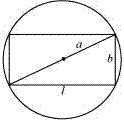Now, by applying the Pythagoras theorem, we have :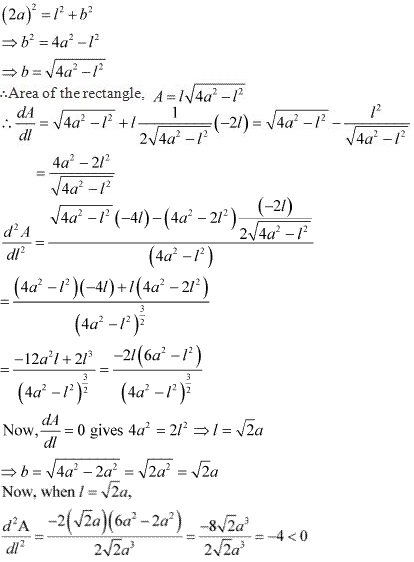∴ By the second derivative test, when l = √2a, then the area of the rectangle is the maximum.
Since  = b =  √2a, the rectangle is a square.
Hence, it has been proved that of all the rectangles inscribed in the given fixed circle, the square has the maximum area.

20. Show that the right circular cylinder of given surface and maximum volume is such that is heights is equal to the diameter of the base.
Solution
Let r and h be the radius and height of the cylinder respectively.
Then, the surface area (S) of the cylinder is given by,
S  = 2πr2 + 2πrh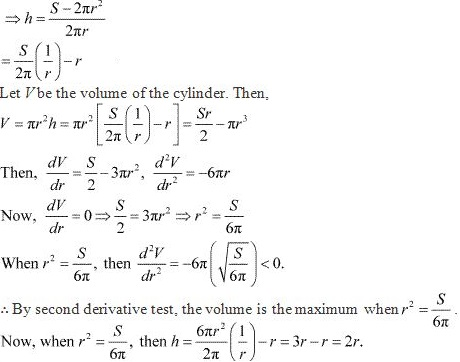Hence, the volume is the maximum when the height is twice the radius i.e., when the height is equal to the diameter.

21. Of all the closed cylindrical cans (right circular), of a given volume of 100 cubic centimetres, find the dimensions of the can which has the minimum surface area?
Solution
Let r and h be the radius and height of the cylinder respectively.
Then, Volume (V) of the cylinder is given by,
V = πr2 h = 100  (given)
∴ h = 100/πr2
Surface area (S) of the cylinder is given by,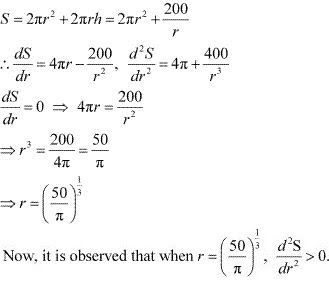∴ By second derivative test, the surface area is the minimum when the radius of the cylinder is (50/π)1/3  cm.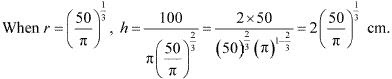Hence, the required dimensions of the can which has the minimum surface area is given by radius = (50/π)1/3 cm and height = 2(50/π)1/3 cm.

22. A wire of length 28 m is to be cut into two pieces. One of the pieces is to be made into a square and the other into a circle. What should be the length of the two pieces so that the combined area of the square and the circle is minimum?
Solution
Let a piece of length l be cut from the given wire to make a square.
Then, the other piece of wire to be made into a circle is of length (28 - l) m.
Now, side of square = l/4.
Let r be the radius of the circle. The, 2πr = 28 - l ⇒ r = 1/2π (28 - l).
The combined areas of the square and the circle (A) is given by,
A = (side of the square)2  + πr2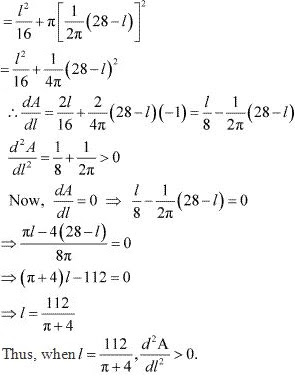∴ By second derivative test, the area (A) is the minimum when l = 112/(π + 4) .
Hence, the combined area is the minimum when the length of the wire in making the square is  112/(π + 4) cm while the length of the wire in making the circle is 28 - 112/(π + 4)  = 28π/(π + 4) cm.

23. Prove that the volume of the largest cone that can be inscribed in a sphere of radius R is 8/27 of the volume of the sphere.
Solution
Let r and h be the radius and height of the cone respectively inscribed in a sphere of radius R.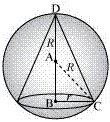Let V be the volume of the cone.
Then, V = (1/3)πr2 h
Height of the cone is given by,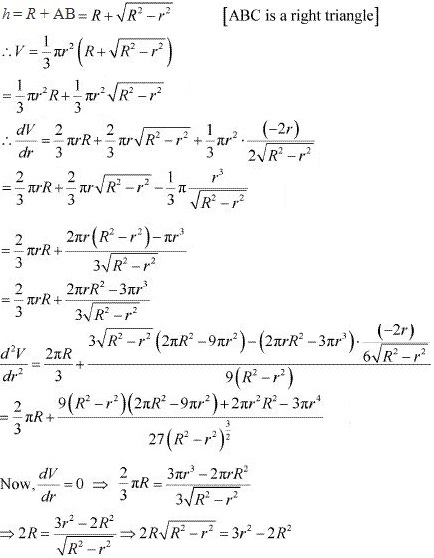⇒ 4R2 (R2 - r2 )= (3r2 - 2R2 )2
⇒ 4R4 - 4R2 r2 = 9r4 + 4R4 - 12r2 R2
⇒ 9r4 = 8R2 r2
⇒ r2 = (8/9)R2
When r2 = (8/9)R2 , then d2 V/dr2  < 0.
∴ By second derivative test, the volume of the cone is the maximum when r2 = (8/9)R2 .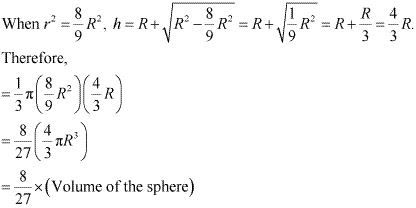Hence, the volume of the largest cone that can be inscribed in the sphere is 8/27
the volume of the sphere.

24. Show that the right circular cone of least curved surface and given volume has an altitude equal to 2 times the radius of the base.
Solution
Let r and h be the radius and the height (altitude) of the cone respectively.
Then, the volume (V) of the cone is given as :
V = (1/3)πr2 h ⇒ h = 3V/πr2
The surface area (S) of the cone is given by,
S = πrl (where l is the slant height )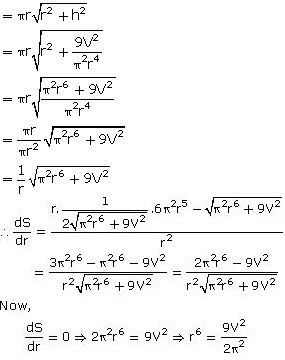Thus, it can be easily verified that when r6 = 9v2/2π2 , d2 s/dr2 > 0
∴ By second derivative test, the surface area of the cone is the least when r6 = 9v2 /2π2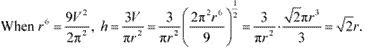Hence, for a given volume, the right circular cone of the least curved surface has an altitude equal to √2 times the radius of the base .

25. Show that the semi-vertical angle of the cone of the maximum volume and of given slant height is tan-1 √2.
Solution
Let θ be the semi - vertical angle of the cone.
It is clear that θ ∊ [0, π/2].
Let r, h, and l be the radius, height, and the slant height of the cone respectively.
The slant height of the cone is given as constant.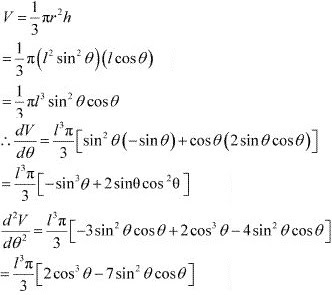Now, dV/dθ = 0
⇒ sin3 θ = 2 sinθ cos2 θ
⇒ tan2 θ = 2
⇒ tan θ = √2
⇒ θ = tan-1 √2
Now, when θ = tan-1 √2, then tan2 θ = 2 or sin2 θ = 2 cos2 θ .
Then, we have :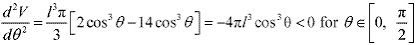∴ By second derivative test, the volume (V) is the maximum when θ = tan-1 √2.
Hence, for a given slant height, the semi - vertical angle of the cone of the maximum volume is tan-1 √2.

26. Show that semi-vertical angle of right circular cone of given surface area and maximum volume is Sin-1 (1/3) .
Solution
Total Surface Area of the cone  = S = πr(l + r) ...(1)
[Where r and l are radius and slant height of the cone respectively]
Volume of cone = V = (1/3)πr2 h
V2 = (1/9)π2 r4 h2
V2 = (1/9)π2 r4 (l2 - r2 )
Using (1), we have
V2 = (1/9)π2 r4 [(s/πr - r)2 - r2 ]
Then by solving further we get -
V2 = (1/9) [S(S r2 - 2πr4 )]
P = V2
differentiating P with respect to r we gwt
dp/dr = (1/9)[S (2Sr - 8πr3 )]
equate  dp/dr = 0
S = 4πr2
Differentiating again with respect to r we find d2 P/dr2 < 0 therefore P is  maximum when S = 4πr2
Differentiating again with respect to r we find that d2 p/dr2  < 0 therefore P is maximum when S = 4πr2
Again therefore, V is maximum when S = 4πr2
πr(l + r) = 4πr2
Thus l = 3r
sin θ = r/l = 1/3
θ = sin-1 (1/3)

27. The point on the curve x2 = 2y which is nearest to the point (0, 5) is
(A) (2√2,4)
(B) (2√2,0)
(C) (0, 0)
(D) (2, 2)
Solution
The given curve is x2 = 2y.
For each value of x, the position of the point will be (x, x2 /2).
The distance d(x) between the points (x, x2 /2) and (0, 5) is given by,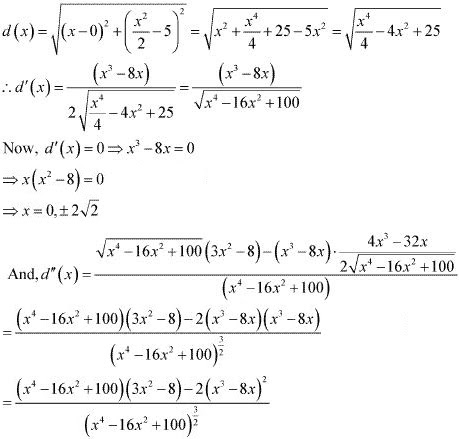When, x = 0 , then d" (x) = 36(-8)/63 < 0.
When, x = ± 2√2, d"(x) > 0.
∴ By second derivative test, d(x) is the minimum at x = ± 2√2.
When x = ± 2√2, y = (2√2)2 /2 = 4.
Hence, the point on the curve x2 = 2y which is nearest to the point (0, 5) is (  ± 2√2, 4).

28. For all real values of x, the minimum value of (1 - x + x2 )/(1 + x + x2 ) is
(A) 0
(B) 1
(C) 3
(D)1/3
Solution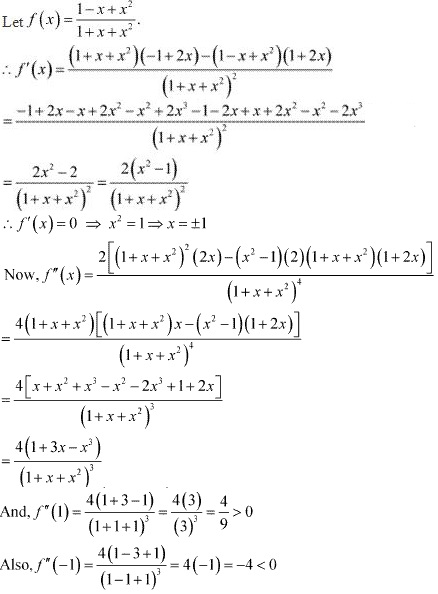∴ By second derivative test, f is the minimum at x = 1 and the minimum value is given by f(1) = (1 - 1 + 1)/(1 + 1 + 1) = 1/3.

29. The maximum value of [x(x - 1) + 1]1/3 , 0 ≤ x ≤ 1 is
(A) (1/3)1/3
(B) 1/2
(C) 1
(D) 0
Solution
Let f(x) = [x(x - 1) + 1]1/3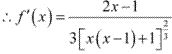Now, f '(x) = 0 ⇒ x = 1/2
Then, we evaluate the value of f at critical point x = 1/2 and at the end points of the interval [0, 1]
{i.e., at x = 0 and x = 1}.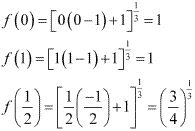Hence, we can conclude that the maximum value of f in the interval [0, 1] is 1.